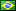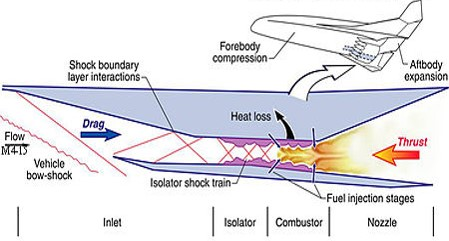aerospace@aerothermalsolutions.co# SCRAMJET – Supersonic Combustion Ramjet## Compartilhar

The following post  is a work from the Unconventional Aerospace Propulsion discipline, carried out by a student at the Federal University of ABC, Caio Fuzaro Rafael, taught by professor Annibal Hetem Jr.

Introduction

Scramjet engines are types of internally propelled engines that can run on various types of chemical fuel combinations. The start of operation of a Scramjet necessarily depends on a flight condition with a high Mach number, commonly these are transported by rockets, and when a high speed is acquired, the engine is started.

Scramjet.

The initial calculations for the design of a Scramjet, take into account supersonic aerodynamics, mainly with regard to the calculation of oblique shock waves.

Shock Waves.

Oblique shock wave equations are easy to solve and can be solved with the help of a graph or table, knowing the Mach number upstream of the slope slope that will deflect the shock wave, and the slope of the slope itself, is simple to obtain the angle of deflection of the shock wave.

Oblique Shock

Oblique Shock

objective

The present work aims to evaluate the geometry proposed by Bonelli (2011) in his paper “Preliminary Design of a Hypersonic Air-breathing Vehicle” presented at the 17th AIAA “International Space Planes and Hypersonic Systems and Technologies Conference”.

Geometry Proposed by Bonnelli (2011)

The proposed geometry was constructed in order to direct the shock waves into the supersonic combustion chamber.

Geometria Scramjet

Details Points 3 and 4

Proposed Geometry

Observações:

• Between points 3 and 4 is located the beginning of the combustion chamber;
• From point 4 and downstream, a thin 1mm thick wall was built to delimit the lower part of the combustion chamber, which runs to the end of the Scramjet’s length.

Preliminary Studies of Geometry and Mach Values

First ramp, between points 1 and 2:

• Ramp inclination angle = 5.62°
• Assuming an input Mach of 7.5 from oblique shock wave accounts we have that Mach number behind the wave will be 6.36 and the wave deflection angle will be 11.82°;
• And yet the ratio between P2/P1 = 2.58 and T2/T1 = 1.34.

Second ramp, between points 2 and 3:

• Angle of inclination between the line passing through points 1 and 2 and the line passing through points 2 and 3 = 13.24°;
• Assuming an input Mach of 6.36 from the oblique shock wave accounts we have that the Mach number behind the wave will be 4.39 and the deflection angle will be 20.36°;
• And yet the ratio between P2/P1 = 5.54 and T2/T1 = 1.86.

Expected results

We can anticipate our results that will be obtained in CFD++:

Expected results.

Mesh for CFD++

A mesh was made with approximately using the software MIME, with 1 million triangular elements, and 400 thousand prism elements that are located in all wall regions of the geometry.

Boundary Conditions

The following boundary conditions were admitted

Boundary Conditions

Rotated Cases

The CFD++ software was used with the following turbulence models:

1.Turbulence Model κ–ε:

• It involves two differential transport equations, is accurate and has numerical stability;
• κ is the kinetic energy of turbulence and is defined as the change in velocity fluctuations;
• ε is the turbulence dissipation rate;
• This model assumes that the flow is turbulent in all its domains.

2.Model with Euler’s Equations:

• Model without viscosity, which solves the Euler equations, which is nothing more than the Navier-Stokes equations without viscous terms.

Turbulence Model κ–ε

Temperature and Pressure Fields.

Mach number and Pressure field.

Mach number.

Recirculation bubble at the inlet of the combustion chamber.

Model with Euler’s Equations

Mach Number and Pressure Fields.

Temperature and Pressure Fields.

Pressure Field inside the Combustion Chamber.

Absence of recirculation bubble at the inlet of the combustion chamber

Mach number

Comparisons and Conclusions:

The graph shown,  presents discontinuities along its curves, these discontinuities are fundamental evidence of shock waves, especially along the combustion chamber.

The table confirms the validation of the designed geometry with the results obtained via CFD.

Pressure distribution along the walls of the Scramjet.

Comparison of obtained and expected results.

The simulated model with k-eps turbulence, points to a recirculation bubble at the entrance of the combustion chamber, it is very likely that this bubble does not exist in reality, it is only a result of the turbulence model, this turbulence model has not been tested in extreme conditions such as altitudes of 30 km with pressure of 1196 Pa and 226 K, so there is no way to say that this is the appropriate turbulence model to simulate, so we chose to run a model without turbulence;

References:

1. 17th AIAA International Space Planes and Hypersonic Systems and Technologies Conference “Preliminary Design of a Hypersonic Air-breathing Vehicle”, Bonelli, F.
2. Modern Compressible Flow: With Historical Perspective, John David Anderson, 3rd. ed., Boston: McGraw-Hill, 2003.
3. Advanced Space Propulsion Systems, Martin Tajmar, Springer; 1st ed., 2004.